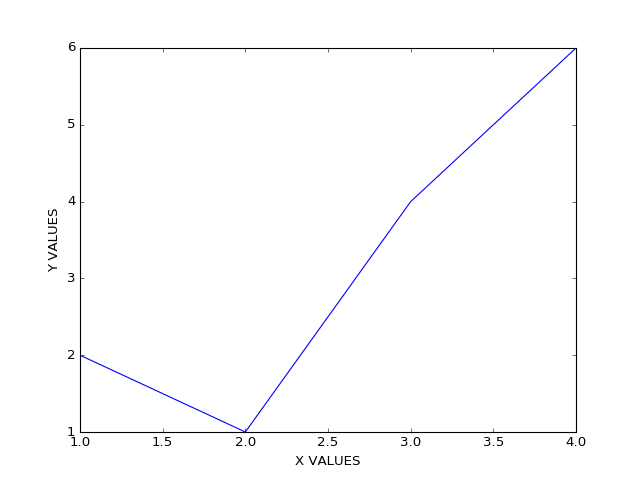I was playing around with Jupyter, namely its feature that allows exporting as a markdown file, and since this site is built with Hugo, a static HTML generator that translates markdown, I thought I’d see what the results looked like.

# Test of Jupyter to Markdown to HTML

## Functional Programming Test

``````a = [1, 2, 3]
b = [1, 2, 3]
c = map(lambda x,y:(x+y)**2/y, a, b)
print "After map: ", c
d = filter(lambda x:x>7, c)
print "After filter: ", d
sum = reduce(lambda x,y:x+y, d)
print "Sum: ", sum
``````
``````After map:  [4, 8, 12]
After filter:  [8, 12]
Sum:  20
``````

## Set Uniqueness Test

``````b = set()
for i in b:
print i
``````
``````1
2
3
``````

## Plot Test

``````#%matplotlib inline
%matplotlib nbagg
import matplotlib.pyplot as plt
plt.plot([1,2,3,4],[2,1,4,6])
plt.ylabel('Y VALUES')
plt.xlabel('X VALUES')
plt.show()
``````Tags: Jupyter
Part of a series on Jupyter.